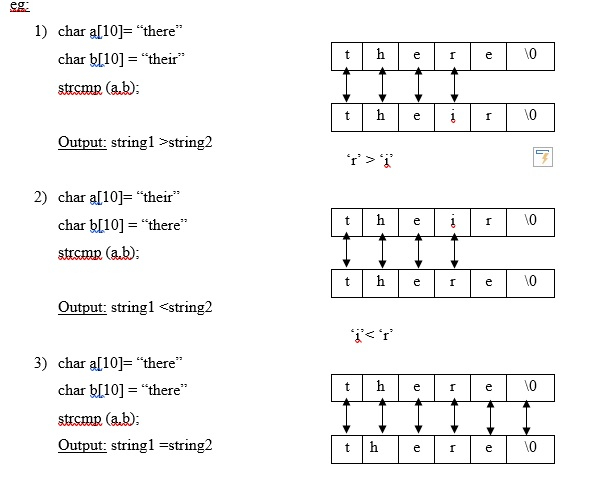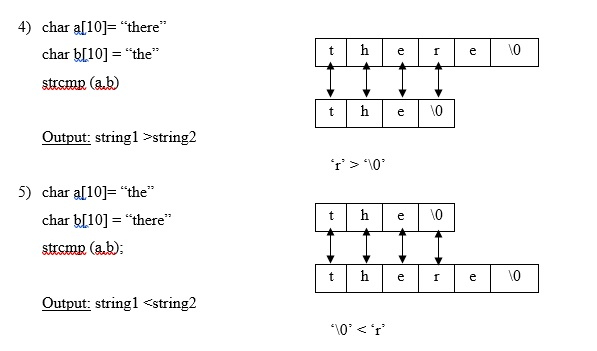# What is strcmp() Function in C language?

The C library function int strcmp(const char *str1, const char *str2) compares the string pointed to, by str1 to the string pointed to by str2.

An array of characters is called a string.

## Declaration

Following is the declaration for an array −

char stringname [size];

For example − char string; string of length 50 characters

## Initialization

• Using single character constant −
char string = { ‘H’, ‘e’, ‘l’, ‘l’, ‘o’ ,‘\0’}
• Using string constants −
char string = "Hello":;

Accessing − There is a control string "%s" used for accessing the string till it encounters ‘\0’.

## The strcmp() function

• This function compares two strings.

• It returns the ASCII difference of the first two non – matching characters in both the strings.

### String comparison

The syntax is as follows −

int strcmp (string1, string2);

If the difference is equal to zero ------ string1 = string2

If the difference is positive ------- string1> string2

If the difference is negative ------- string1 <string2## Example Program

The following program shows the usage of strcmp() function −

Live Demo

#include<stdio.h>
main ( ){
char a, b ;
int d;
printf ("enter 2 strings:");
scanf ("%s %s", a,b);
d = strcmp (a,b);
if (d==0)
printf("%s is equal to %s", a,b);
else if (d>0)
printf("%s is greater than %s",a,b);
else if (d<0)
printf("%s is less than %s", a,b);
}

## Output

When the above program is executed, it produces the following result −

enter 2 strings:
bhanu
priya
bhanu is less than priya

Let’s see another example on strcmp().

Given below is the C program to compare two strings using strcmp library function −

## Example

Live Demo

#include<stdio.h>
#include<string.h>
void main(){
//Declaring two strings//
char string1,string2;
int value;
//Reading string 1 and String 2//
printf("Enter String 1: ");
gets(string1);
printf("Enter String 2: ");
gets(string2);
//Comparing using library function//
value = strcmp(string1,string2);
//If conditions//
if(value==0){
printf("%s is same as %s",string1,string2);
}
else if(value>0){
printf("%s is greater than %s",string1,string2);
}
else{
printf("%s is less than %s",string1,string2);
}
}

## Output

When the above program is executed, it produces the following result −

Enter String 1: Tutorials
Enter String 2: Point
Tutorials is greater than Point